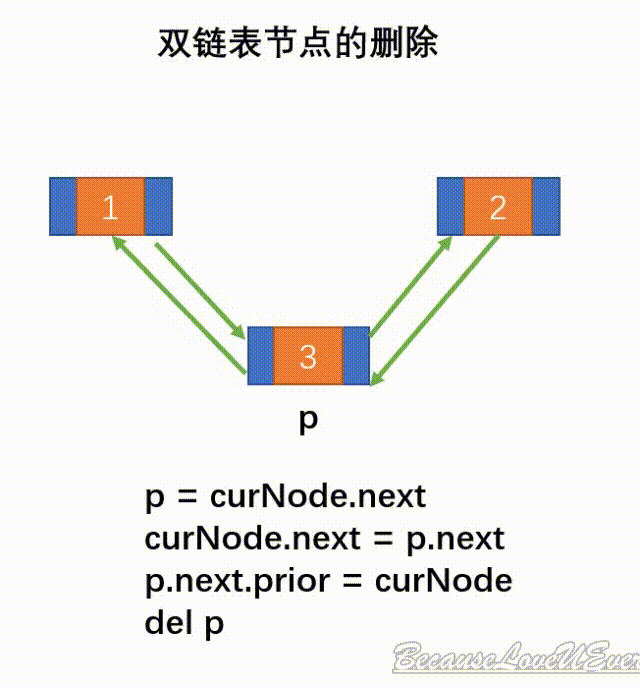# 结构python

## 2.在顺序存储结构上 利用Python语言将顺序表中的元素倒置

#-*- coding utf-8 -*-__author__="clearlove"class SeqList(object)：#创建8个元素大小的列表 def __init__(self,max=10): self.max=max #创建默认为8 self.num=0 self.date=[None]*self.max def is_empty(self): return self.num is 0 def is_full(self): return self.num is self.max def __getitem__(self,key)：#获取某个位置的元素 if not isinstance(key,int): raise TypeError if 0<=keyself.num: raise IndexError for j in range(self.num,key,-1): self.date[j]=self.date[j-1] self.date[key]=value self.num+=1 def remove(self,key): if not isinstance(key,int): raise TypeError if key<0 or key>self.num: raise IndexError for j in range(key,self.num): self.date[j]=self.date[j+1] self.num-=1 #输出操作 def printList(self): for i in range(0,self.num): print (self.date[i]) #清除操作 def clear(self): self.__init__()if __name__=="__main__": a=SeqList() print(a.date) #num=0 print(a.is_empty()) a.append(0) a.append(1) a.append(2) print (a.date) print (a.num) print (a.max) a.insert(1,6) print (a.date) a.append(8) print (a.date) a.printList() a.clear()。

## 3.python中什么数据结构 index

def tree_find(tree, value):

def tree_rec(tree, iseq):

if isinstance(tree, list):

for i, child in enumerate(tree):

r = tree_rec(child, iseq + [i])

if r is not None:

return r

elif tree == value:

return iseq

else:

return None